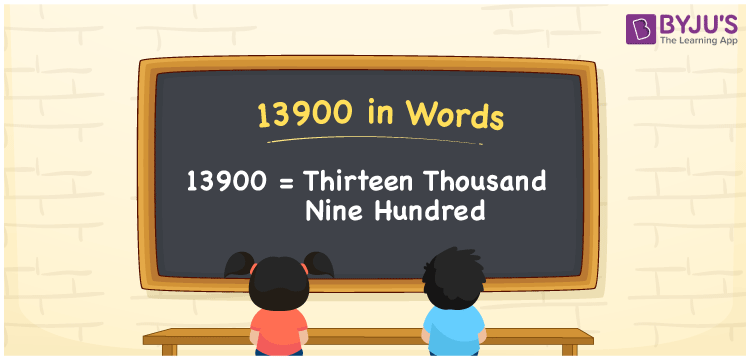# 13900 in Words

13900 in words is written as Thirteen thousand nine hundred. In both the International System of Numerals and the Indian System of Numerals, 13900 in words is written as Thirteen thousand nine hundred. The number 13900 is a Cardinal Number as it represents some quantity. For example, “this mobile phone costs 13900 rupees”, 13900 quantifies the cost of something.

 13900 in words Thirteen thousand nine hundred Thirteen thousand nine hundred in number 13900

## 13900 in English Words

13900 in English words is read as “Thirteen thousand nine hundred.”## How to Write 13900 in Words?

To write 13900 in words, we shall use the place value chart. In the place value chart, put 1 in the ten thousands, 3 in the thousands, 9 in the hundreds, and 0 in the tens and the ones, respectively. Let us make a place value chart to write the number 13900 in words.

 Ten Thousands Thousands Hundreds Tens Ones 1 3 9 0 0

Thus, we can write the expanded form as

1 × Ten Thousand + 3 × Thousand + 9 × Hundred + 0 × Ten + 0 × One

= 1 × 10000 + 3 × 1000 + 9 × 100 + 0 × 10 + 0 × 1

= 10000 + 3000 + 900 + 0 + 0

= 13900

= Thirteen thousand nine hundred.

13900 is the natural number that is succeeded by 13899 and preceded by 13901.

13900 in words – Thirteen thousand nine hundred

• Is 13900 an odd number? – No
• Is 13900 an even number? – Yes
• Is 13900 a perfect square number? – No
• Is 13900 a perfect cube number? – No
• Is 13900 a prime number? – No
• Is 13900 a composite number? – Yes

## Frequently Asked Questions on 13900 in Words

### How to write 13900 in words?

13900 in words is written as Thirteen thousand nine hundred.

### How to write 13900 in words in the International and Indian System of Numerals?

In both, the system of numerals, 13900 in words, is written as Thirteen thousand nine hundred.

### How to write 13900 in a place value chart?

In the place value chart, write 1 in the ten thousands, 3 in the thousands, 9 in the hundreds, and 0 in the tens and ones, respectively.